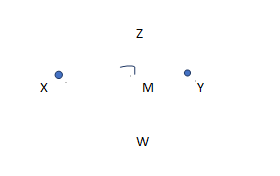Perpendicular Bisector

Perpendicular Bisector of a Line Segment

Before we understand, what is perpendicular bisector, let us go through the basics required to understand perpendicular bisector.
A circle, unlike the other geometric figures, has no straight lines. It is a blend of curves all connected. There are no angles to be seen in a circle. A rectangle is formed by connecting four lines segments, in a related manner to a square. Nevertheless, a rectangle will typically have two-line segments which are longer than the other two-line segments. It could be represented as an elongated square. Again, in a rectangle, the four corners are formed to form four right angles.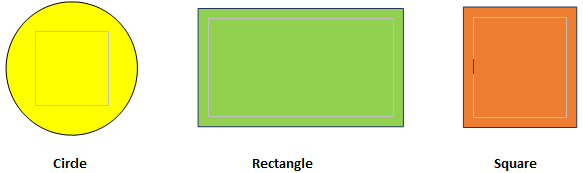Circles

A circle is the set of every point in the plane that is the same distance away from a specific point, called the center. The center of the circle here is point A. We tell this circle “circle A,” and it is labeled ⨀A.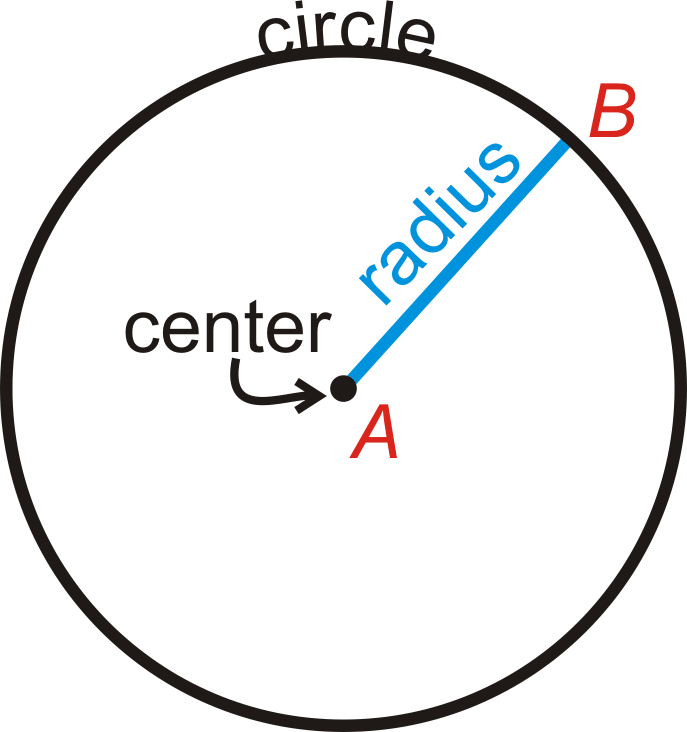Circles

Some Important parts of the circle

• A Radius is a distance from the center of the circle to its outer side.

• A Chord is a line segment where the endpoints are on a circle.

• A chord is that which passes through the center of the circle is called a diameter

•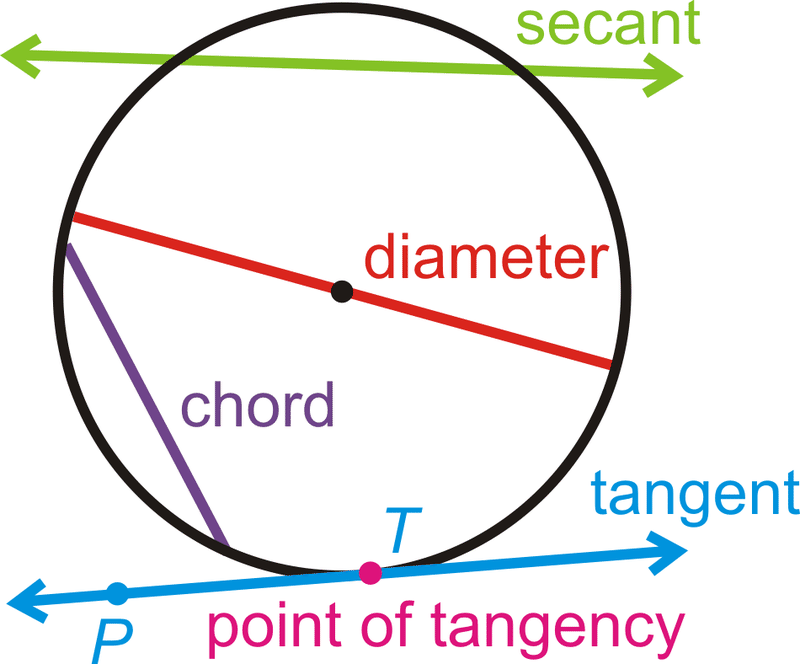• . The length of a diameter is 2 times the length of a radius such that d = 2r.

• A line that intersects a circle in 2 points is called secant.

• A line that intersects a circle in exactly 1 point is called a tangent.

• A Point of Tangency is the point where a tangent line touches the circle.

• A triangle consists of three lines of segments combined. Unlike a square and a rectangle, the angles in a triangle can be of multiple measurements and are not always right angles. Triangles often take their name from the type of angles which can be observed within the triangle itself. A triangle which has one right angle can be related to as a right triangle. An acute triangle is formed when all of the angles within the triangle measure less than ninety degrees. An obtuse triangle is formed when one angle within the triangle measures more than ninety degrees. Lastly, an equiangular triangle is created when all of the angles within the triangle measure sixty degrees. Additionally, triangles can be labeled or distinguished by the type of sides they may have. There are no congruent sides in a scalene triangle. There are 2 congruent sides in an isosceles triangle. In an equilateral triangle, there are three congruent sides. Equiangular and equilateral triangles are two distinct terms for the same triangle.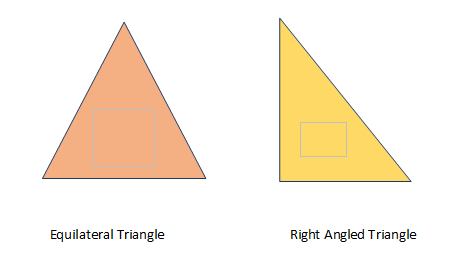A parallelogram is called a parallelogram because the opposite sides of the shape are parallel. In order to determine if the sides can be said parallel, one must examine them closely. Parallel lines will never intersect, or cross, no matter how long they are stretched. Therefore, in a shape, if one visualizes the lines extended and extended through eternity and they never will meet or touch, then they can be viewed a parallel. However, if the lines meet or touch, they cannot be considered parallel and the shape could not then be called a parallelogram. In this form, the shape of a triangle cannot be considered a parallelogram, as the two lines opposite of each other meet at the point of the triangle. Since the lines meet, they are not parallel.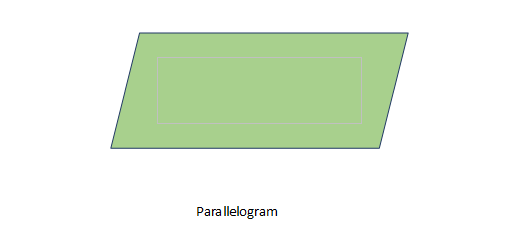What is perpendicular bisector?
To understand this let us take a closer look into these two words. First, let us understand what does perpendicular mean.

Perpendicular, are the lines, rays, the line segment that intersects each other to form right angles. The symbol for the perpendicular is ( ). Let us take a look at few examples say we have two lines as shown below these two lines are perpendicular if they intersect to form a right angle.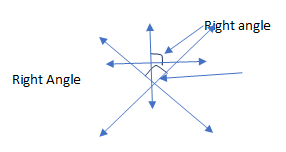Now let us take a look at the second word Bisector. We know the general meaning for the word bisect which means to cut something into half so a Bisector is a line, ray or another figure that cut them into two congruent (they intersect at the same point ) pieces.

Let us take a look at few examples, let us say we have a segment AB, and we have another line which bisects segment AB at D which means that segment AD is congruent to segment DB and we can say that line CD is a bisector of segment AB.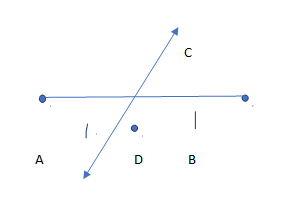Let us consider an angle EFG, we can say that ray FH is a bisector for angle EFG hence angle EFH is congruent to angle GFH.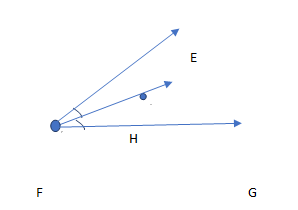Now we have understood the meaning of both perpendicular and bisector. Now let us define Perpendicular bisector “They have a line segment that is perpendicular to a line and cuts the segment into 2 congruent pieces.”

Say we have a line segment XY and then we have line perpendicular to the line segment and divides the segment into two congruent segments. We can see that segment XM is congruent to segment MY. We can conclude that line ZW is a perpendicular bisector for the line segment XY. Notice that point M is the mid-point of the line segment.